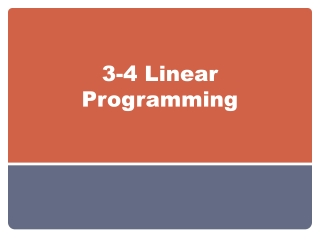DownloadDownload Presentation3-4 Linear Programming

# 3-4 Linear Programming

Télécharger la présentation## 3-4 Linear Programming

- - - - - - - - - - - - - - - - - - - - - - - - - - - E N D - - - - - - - - - - - - - - - - - - - - - - - - - - -
##### Presentation Transcript

1. 3-4 Linear Programming

2. Adjusting the Window To adjust the viewing window, press window Xmin = smallest x-value Xmax = largest x-value Xscl = # of units represented by tick marks on the x-axis Ymin= smallest y-value Ymax= largest y-value Yscl= # of units represented by tick marks on the y-axis

3. y – x + yx + y 3x – 11 2 3 11 3 < 1 4 11 4 > > Step 1:Graph the constraints. 2 3 11 3 y = – x + y = x + To find A, solve the system . The solution is (1, 3), so A is at (1, 3). 11 4 1 4 11 4 1 4 y = x + y = 3x – 11 To find B, solve the system . The solution is (5, 4), so B is at (5, 4). Linear Programming Lesson 3-4 Additional Examples Find the values of x and y that maximize and minimize P if P = –5x + 4y. Step 2: Find the coordinates for each vertex.

4. 2 3 11 3 y = – x + y = 3x – 11 To find C, solve the system . The solution is (4, 1), so C is at (4, 1). Linear Programming Lesson 3-4 Additional Examples (continued) Step 3: Evaluate P at each vertex. Vertex P = –5x + 4y A(1, 3) P = –5(1) + 4(3) = 7 B(5, 4) P = –5(5) + 4(4) = –9 C(4, 1) P = –5(4) + 4(1) = –16 When x = 1 and y = 3, P has its maximum value of 7. When x = 4 and y = 1, P has its minimum value of –16.

5. Define:Let x = number of tables made in a day. Let y = number of chairs made in a day. Let P = total profit. Relate:  Organize the information in a table. Tables Chairs Total No. of Products xyx + y No. of Units 30 x 60 40 y 100 120 Profit 150x 65y 150x + 65y < < < < constraint objective Linear Programming Lesson 3-4 Additional Examples A furniture manufacturer can make from 30 to 60 tables a day and from 40 to 100 chairs a day. It can make at most 120 units in one day. The profit on a table is \$150, and the profit on a chair is \$65. How many tables and chairs should they make per day to maximize profit? How much is the maximum profit?

6. > < > < < Step 1: Graph the constraints. Linear Programming Lesson 3-4 Additional Examples (continued) x 30 x 60 y 40 y 100 x + y 120 Write: Write the constraints. Write the objective function. P = 150x + 65y Step 2: Find the coordinates of each vertex. Vertex A(30, 90) B(60, 60) C(60, 40) D(30, 40) Step 3: Evaluate P at each vertex. P = 150x + 65y P = 150(30) + 65(90) = 10,350 P = 150(60) + 65(60) = 12,900 P = 150(60) + 65(40) = 11,600 P = 150(30) + 65(40) = 7100 The furniture manufacturer can maximize their profit by making 60 tables and 60 chairs. The maximum profit is \$12,900.